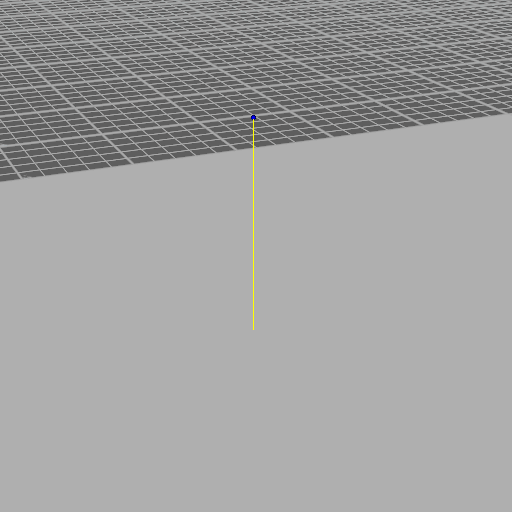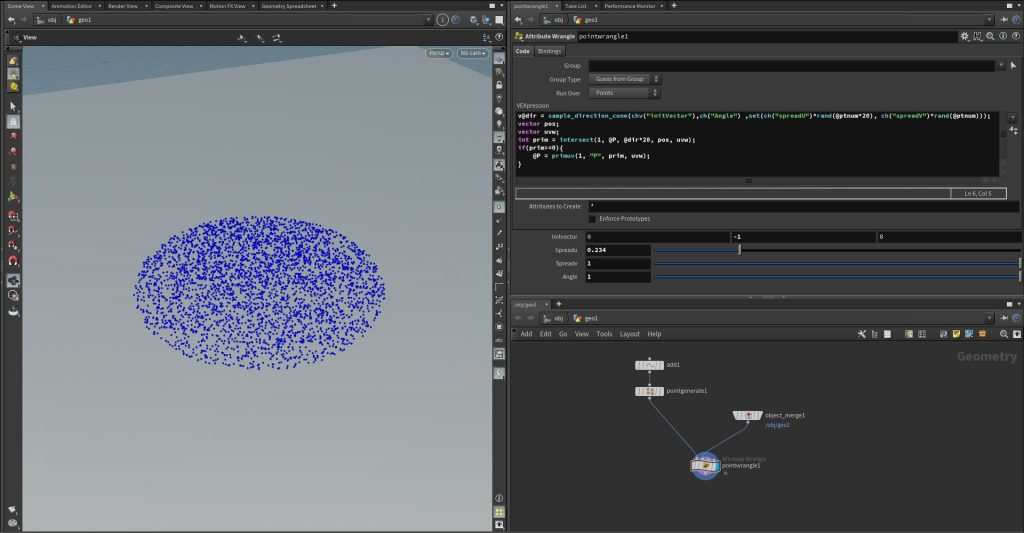## Function of the Week: sample_direction_cone

Hey,

today I’ll establish my personal Node/Function of the week. This week it’s the vex function “sample_direction_cone”.

The help says: “Returns a unit vector, i.e. a vector of length 1, based on u. Given uniform random u pairs of values in [0,1), the returned unit vectors will be uniform random and continuous with respect to u on the surface of the unit sphere, in the area within maxangle of the direction indicated by center.”

So what to use this for? My usual cases are randomization of velocity vectors, ray cast operations or additive spread-transforms of scattered pieces. XSI operators will know this kind of function from the ICE function “randomize vector by cone” – This is the equal Houdini function.Here is an point wrangle example, that demonstrates the function in combination with an raycast function.

```v@dir = sample_direction_cone(chv("initVector"),ch("Angle") ,set(ch("spreadU")*rand(@ptnum*20), ch("spreadV")*rand(@ptnum))); vector pos; vector uvw; int prim = intersect(1, @P, @dir*20, pos, uvw); if(prim>=0){ @P = primuv(1, "P", prim, uvw); }```